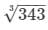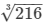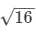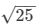# RD Sharma Solutions - Chapter 21 - Mensuration - II (Part - 3), Class 8, Maths Notes | Study RD Sharma Solutions for Class 8 Mathematics - Class 8

## Class 8: RD Sharma Solutions - Chapter 21 - Mensuration - II (Part - 3), Class 8, Maths Notes | Study RD Sharma Solutions for Class 8 Mathematics - Class 8

The document RD Sharma Solutions - Chapter 21 - Mensuration - II (Part - 3), Class 8, Maths Notes | Study RD Sharma Solutions for Class 8 Mathematics - Class 8 is a part of the Class 8 Course RD Sharma Solutions for Class 8 Mathematics.
All you need of Class 8 at this link: Class 8

PAGE NO 21.22:

Question 1:

Find the surface area of a cuboid whose
(i) length = 10 cm, breadth = 12 cm, height = 14 cm
(ii) length = 6 dm, breadth = 8 dm, height = 10 dm
(iii) length = 2 m, breadth = 4 m, height = 5 m
(iv) length = 3.2 m, breadth = 30 dm, height = 250 cm.

(i)Dimension of the cuboid:Length = 10 cm

Height = 14 cm

Surface area of the cuboid = 2 × (length × breadth + breadth × height + length × height)

= 2 × (10 × 12 + 12 × 14 + 10 × 14) = 2 × (120 + 168 + 140) = 856 cm²

(ii)Dimensions of the cuboid:Length = 6 dm

Height = 10 dm

Surface area of the cuboid = 2 × (length × breadth + breadth × height + length × height)

= 2 × (6 × 8 + 8 × 10 + 6 × 10)

= 2 × (48 + 80 + 60) = 376 dm²

(iii) Dimensions of the cuboid:

Length = 2 m Breadth = 4 m

Height = 5 m

Surface area of the cuboid = 2 × (length × breadth + breadth × height + length × height)

= 2 × (2 × 4 + 4 × 5 + 2 × 5) = 2 × (8 + 20 + 10) = 76 m²

(iv)Dimensions of the cuboid:

Length = 3.2m

= 3.2 × 10 dm  (1 m = 10 dm)

= 32 dm

Height = 250 cm

= 250 × 1/10dm   (10cm  =  1 dm)

= 25 dm

Surface area of the cuboid = 2 × (length × breadth + breadth × height + length × height)

= 2 × (32 × 30 + 30 × 25 + 32 × 25)

= 2 × (960 + 750 + 800) = 5020 dm²

Question 2:

Find the surface area of a cube whose edge is
(i) 1.2 m
(ii) 27 cm
(iii) 3 cm
(iv) 6 m
(v) 2.1 m

(i) Edge of the a cube = 1.2 m

∴ Surface area of the cube = 6 × (side)2 = 6 × (1.2)2 = 6 × 1.44 = 8.64 m².

(ii) Edge of the a cube = 27 cm

∴ Surface area of the cube = 6 × (side)2 = 6 × (27)2 = 6 × 729 = 4374 cm²

(iii) Edge of the a cube = 3 cm

∴ Surface area of the cube = 6 × (side)2 = 6 × (3)= 6 × 9 = 54 cm²

(iv) Edge of the a cube = 6 m

∴ Surface area of the cube = 6 × (side)= 6 × (6)= 6 × 36 = 216 m²

(v) Edge of the a cube = 2.1 m

∴ Surface area of the cube = 6 × (side)2 = 6 × (2.1)2 = 6 × 4.41 = 26.46 m²

Question 3:

A cuboidal box is 5 cm by 5 cm by 4 cm. Find its surface area.

The dimensions of the cuboidal box are 5 cm × 5 cm × 4 cm.

Surface area of the cuboidal box = 2 × (length × breadth + breadth × height + length × height)

= 2 × (5 × 5 + 5 × 4 + 5 × 4)

= 2 × (25 + 20 + 20) = 130 cm²

Question 4:

Find the surface area of a cube whose volume is
(i) 343 m3
(ii) 216 dm3

(i)Volume of the given cube = 343 m

We know that volume of a cube = (side)3

⇒ (side)3 = 343  i.e., side  == 7 m

∴ Surface area of the cube = 6 × (side)2 = 6 × (7)2 = 294 m²

(ii)Volume of the given cube = 216 dm3

We know that volume of a cube = (side)3

⇒ (side)3 = 216 i.e., side == 6 dm

∴ Surface area of the cube = 6 × (side)2 = 6 × (6)2 = 216 dm²

Question 5:

Find the volume of a cube whose surface area is
(i) 96 cm²
(ii) 150 m²

(i)Surface area of the given cube = 96 cm²

Surface area of a cube = 6 × (side)2

⇒ 6 × (side)2 = 96

⇒ (side)2 = 96/6 = 16 i.e., side of the cube == 4 cm

∴ Volume of the cube = (side)3 = (4)3 = 64 cm3

(ii)Surface area of the given cube = 150 m²

Surface area of a cube = 6 × (side)2

⇒ 6 × (side)2 = 150

⇒ (side)= 150/6 = 25

i.e., side of the cube == 5 m

∴ Volume of the cube = (side)3 = (5)3 = 125 m3

Question 6:

The dimensions of a cuboid are in the ratio 5 : 3 : 1 and its total surface area is 414 m². Find the dimensions.

It is given that the sides of the cuboid are in the ratio 5:3:1.

Suppose that its sides are x multiple of each other, then we have:Length = 5x m

Height = x m

Also, total surface area of the cuboid = 414 m²

Surface area of the cuboid = 2 × (length × breadth + breadth × height + length × height)

⇒ 414 = 2 × (5x × 3x + 3x × 1x + 5x × x)

⇒ 414 = 2 × (15x+ 3x2 + 5x2

⇒ 414 = 2 × (23x2

⇒ 2 × (23 × x2) = 414

⇒ (23 × x2) = 414/2 = 207

⇒ x2 = 207/23 = 9

⇒ x = √9 = 3

Therefore, we have the following:

Lenght of the cuboid = 5 × x = 5 × 3 = 15 m

Breadth of the cuboid = 3 × x = 3 × 3 = 9 m

Height of the cuboid = x = 1 × 3 = 3 m

Question 7:

Find the area of the cardboard required to make a closed box of length 25 cm, 0.5 m and height 15 cm.

Length of the box = 25 cm

Width of the box = 0.5 m

= 0.5 × 100 cm  (∵ 1 m =  100 cm)

= 50 cm

Height of the box = 15 cm

∴ Surface area of the box = 2 × (length × breadth + breadth × height + length × height)

= 2 × (25 × 50 + 50 × 15 + 25 × 15)

= 2 × (1250 + 750 + 375)

= 4750 cm²

Question 8:

Find the surface area of a wooden box whose shape is of a cube, and if the edge of the box is 12 cm.

It is given that the side of the cubical wooden box is 12 cm.

∴ Surface area of the cubical box = 6 × (side)2 = 6 × (12)2

= 864 cm²

It is given that the side of the cubical wooden box is 12 cm.

∴ Surface area of the cubical box = 6 × (side)2 = 6 × (12)2

= 864 cm²

Question 9:

The dimensions of an oil tin are 26 cm × 26 cm × 45 cm. Find the area of the tin sheet required for making 20 such tins. If 1 square metre of the tin sheet costs Rs 10, find the cost of tin sheet used for these 20 tins.

Dimensions of the oil tin are 26 cm × 26 cm × 45 cm.

So, the area of tin sheet required to make one tin = 2 × (length × breadth + breadth × height + length × height)

= 2 × (26 × 26 + 26 × 45 + 26 × 45)

= 2 × (676 + 1170 + 1170)

= 6032 cm²

Now, area of the tin sheet required to make 20  such tins = 20 × surface area of one tin = 20 × 6032 = 120640 cm²

It can be observed that 120640 cm² = 120640 × 1cm × 1cm

= 120640 × 1/100m × 1/100m   (∵ 100 cm = 1 m)

= 12.0640 m²

Also, it is given that the cost of 1 m² of tin sheet = Rs 10

∴ The cost of 12.0640 m² of tin sheet = 12.0640 × 10 = Rs 120.6

Question 10:

A cloassroom is 11 m long, 8 m wide and 5 m high. Find the sum of the areas of its floor and the four walls (including doors, windows, etc.)

Lenght of the classroom = 11 m

Width = 8 m

Height = 5 m

We have to find the sum of the areas of its floor and the four walls (i.e., like an open box).

∴ The sum of areas of the floor and the four walls = (length × width) + 2 × (width × height + length × height)

= (11 × 8) + 2 × (8 × 5 + 11 × 5)

= 88 + 2 × (40 + 55)

= 88 + 190 = 278 m²

Question 11:

A swimming pool is 20 m long 15 m wide and 3 m deep. Find the cost of repairing the floor and wall at the rate of Rs 25 per square metre.

Length of the swimming pool = 20 m

Height = 3 m

Now, surface area of the floor and all four walls of the pool = (length × breadth) + 2 × (breadth × height + length × height)

= (20 × 15) + 2 × (15 × 3 + 20 × 3)

= 300 + 2 × (45 + 60)

= 300 + 210 = 510 m²

The cost of repairing the floor and the walls is Rs 25/m².

∴ The total cost of repairing 510 m² area = 510 × 25 = Rs 12750

Question 12:

The perimeter of a floor of a room is 30 m and its height is 3 m. Find the area of four walls of the room.

Perimeter of the floor of the room = 30 m

Height of the oom = 3 m

Perimeter of a rectangle = 2 × (length + breadth) = 30 m

So, area of the four walls = 2 × (length × height + breadth × height)

= 2 × (length + breadth) × height = 30 × 3 = 90 m²

Question 13:

Show that the product of the areas of the floor and two adjacent walls of a cuboid is the square of its volume.

Suppose that the length, breadth and height of the cuboidal floor are l cm, b cm and h cm, respectively.Then, area of the floor = l × b cm²

Area of the wall = b × h  cm²

Area of its adjacent wall = l × h cm²

Now, product of the areas of the floor and the two adjacent walls = (l × b) × (b × h) × (l × h) = l × b× h2 = (l × b × h)2

Also, volume of the cuboid = l × b × h cm²

∴ Product of the areas of the floor and the two adjacent walls = (l × b × h)2 = (volume)2

Question 14:

The walls and ceiling of a room are to be plastered. The length, breadth and height of the room are 4.5 m, 3 m and 350 cm, respectively. Find the cost of plastering at the rate of Rs 8 per square metre.

Length of a room = 4.5 m

Height = 350 cm

= 350/100m    (∵ 1 m =  100 cm )

= 3.5 m

Since only the walls and the ceiling of the room are to be plastered, we have:

So, total area to be plastered

= area of the ceiling + area of the walls = (length × breadth) + 2 × (length × height + breadth × height)

= (4.5 × 3) + 2 × (4.5 × 3.5 + 3 × 3.5)

= 13.5 + 2 × (15.75 + 10.5) = 13.5 + 2 × (26.25) = 66 m²

Again, cost of plastering an area of 1 m² = Rs 8

∴ Total cost of plastering an area of 66 m² = 66 × 8 = Rs 528

PAGE NO 21.23:

Question 15:

A cuboid has total surface area of 50 m² and lateral surface area is 30 m². Find the area of its base.

Total surface area of the cuboid = 50 m²

Its lateral surface area = 30 m²

Now, total surface area of the cuboid = 2 × (surface area of the base) + (surface area of the 4 walls)

⇒ 50 = 2 × (surface area of the base) + (30)

⇒ 2 × (surface area of the base) = 50−30 = 20

∴ Surface area of the base = 202 = 10 m²

Total surface area of the cuboid = 50 m²

Its lateral surface area = 30 m²

Now, total surface area of the cuboid = 2 × (surface area of the base) + (surface area of the 4 walls)

⇒ 50 = 2 × (surface area of the base) + (30)

⇒ 2 × (surface area of the base) = 50-30 = 20

∴ Surface area of the base = 20/2 = 10 m²

Question 16:

A classroom is 7 m long, 6 m broad and 3.5 m high. Doors and windows occupy an area of 17 m². What is the cost of white-washing the walls at the rate of Rs 1.50 per m².

Length of the classroom = 7m

Breadth of the classroom = 6 m

Height of the classroom = 3.5 m

Total surface area of the classroom to be whitewashed = areas of the 4 walls

= 2 × (breadth × height + length × height)

= 2 × (6 × 3.5 + 7 × 3.5) = 2 × (21 + 24.5) = 91 m²

Also, the doors and windows occupy 17 m².

So, the remaining area to be whitewashed = 91−17 = 74 m²

Given that the cost of whitewashing 1 m² of wall = Rs 1.50

∴ Total cost of whitewashing 74 m² of area = 74 × 1.50 = Rs 111

Question 17:

The central hall of a school is 80 m long and 8 m high. It has 10 doors each of size 3 m × 1.5 m and 10 windows each of size 1.5 m × 1 m. If the cost of white-washing the walls of the hall at the rate of Rs 1.20 per m² is Rs 2385.60, find the breadth of the hall.

Suppose that the breadth of the hall is b m.

Lenght of the hall = 80 m

Height of the hall = 8 m

Total surface area of 4 walls including doors and windows = 2 × (length × height + breadth × height)

= 2 × (80 × 8 + b × 8)

= 2 × (640 + 8b) = 1280 + 16b m²

The walls have 10 doors each of dimensions 3 m × 1.5 m. i.e., area of a door = 3 × 1.5 = 4.5 m²

∴ Area of 10 doors = 10 × 4.5 = 45 m²

Also, there are 10 windows each of dimensions 1.5 m × 1 m.i.e., area of one window = 1.5 × 1 = 1.5 m²

∴ Area of 10 windows = 10 × 1.5 = 15 m²

Thus, total area to be whitwashed = (total area of 4 walls)−(areas of 10 doors + areas of 10 windows)

= (1280 + 16b)−(45 + 15) = 1280 + 16b−60

= 1220 + 16b m²

It is given that the cost of whitewashing 1 m² of area = Rs 1.20

∴ Total cost of whitewashing the walls = (1220 + 16b) × 1.20

= 1220 × 1.20 + 16b × 1.20

= 1464 + 19.2b

Since the total cost of whitewashing the walls is Rs 2385.60, we have:1464 + 19.2b = 2385.60

⇒ 19.2b = 2385.60−1464

⇒ 19.2b = 921.60

⇒ b = 921.60/19.2 = 48 m

∴ The breadth of the central hall is 48 m.

The document RD Sharma Solutions - Chapter 21 - Mensuration - II (Part - 3), Class 8, Maths Notes | Study RD Sharma Solutions for Class 8 Mathematics - Class 8 is a part of the Class 8 Course RD Sharma Solutions for Class 8 Mathematics.
All you need of Class 8 at this link: Class 8Use Code STAYHOME200 and get INR 200 additional OFF

## RD Sharma Solutions for Class 8 Mathematics

88 docs

Track your progress, build streaks, highlight & save important lessons and more!

,

,

,

,

,

,

,

,

,

,

,

,

,

,

,

,

,

,

,

,

,

,

,

,

,

,

,

;